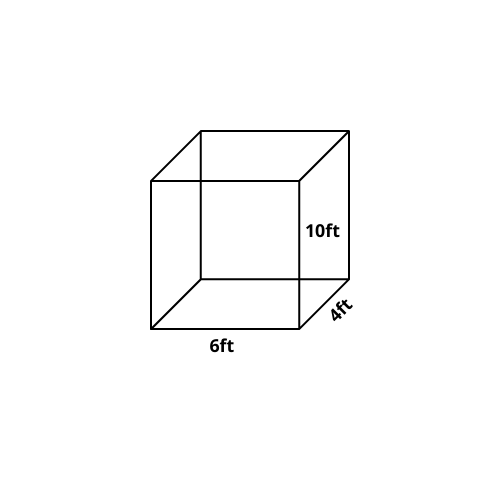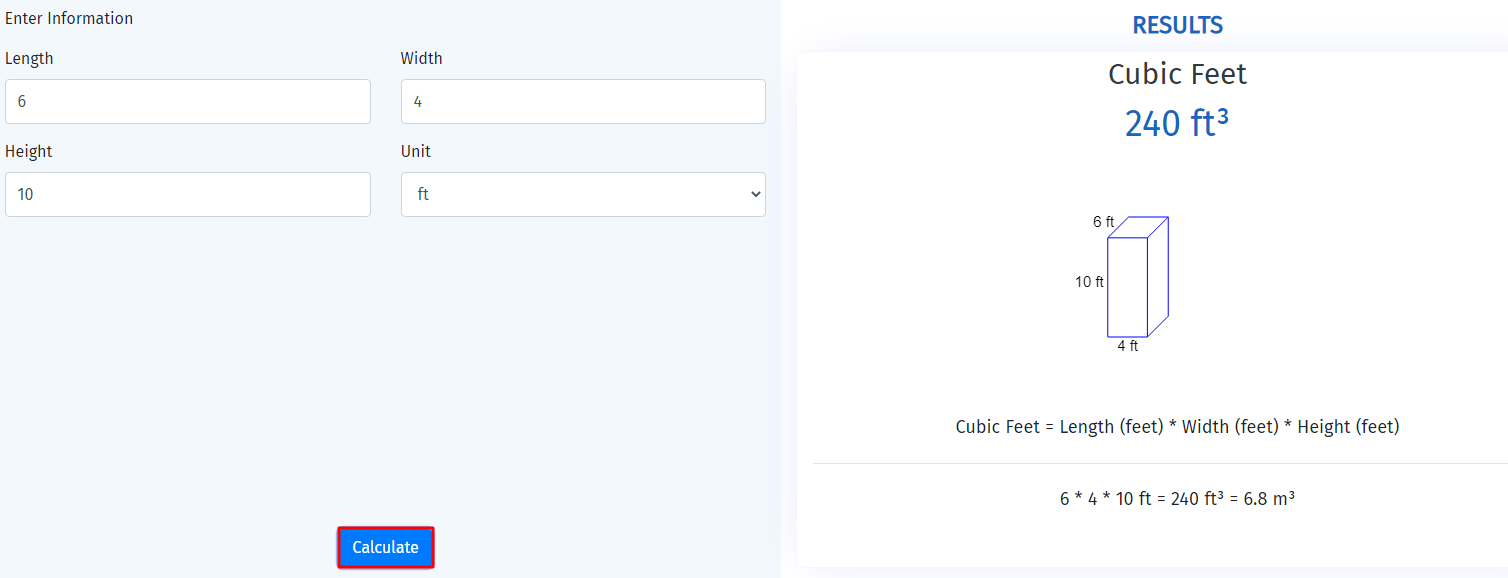# Introduction to cubic feet, its definition, Importance and examples

Shape is one of the oldest branches of mathematics. Shape aids us in determining which materials to utilize and what design to create, as well as playing an important role in the construction process.

It was initially developed as a useful reference for quantifying capacities, dimensions, and areas, and it is still in use today.

The term “cubic” refers to a specific volume obtained by multiplying the dimensions of a tri object. To simply state, the volume of an item is calculated by multiplying its length, breadth, and height.

The feet part is where you start when converting to 3D units. A foot is a 12 inch long imperial unit of measurement, or approximately 30.5 cm.

Instead, multiply the length by the width, then multiply again to obtain the height. I’m rather certain. Cubic feet are used to calculate the volume of any three-dimensional object, such as a cubic tank, cylinder, or pyramid.

Book A Free Demo# What is cubic feet?

A cubic foot (ft3) is a measurement of volume in the US customary and imperial systems of measurement. Single square foot equals the volume of a cube with one-foot-long edges.

Or

Cubic feet is a standard measure that is commonly used to express the volume of three-dimensional geometries with 3 sides.

## Formula of cubic feet

Because cubic feet is a capacity that comprises length, breadth, and height, its formula is as follows:

Cubic feet = Capacity = Volume = L × W × H

Where L represents the shape’s length, W represents width, and H is its height. The above mentioned formula shows that you just have to multiply the three dimensions of a cube to calculate its 3-D capacity. If the number of cubic feet is known, the preceding procedure can be adjusted to obtain its dimensions.

L = Volume / W × H

W = Volume / L × H

H = Volume / L × W

These concepts are quite tricky and if you are looking for guidance then do refer to our online math tutoring classes.

Book A Free Demo

Capacity’s Units

One of the commonly used units of volume is cubic feet. It’s most popular in the US. Although it is used to some extent in Canada and England.

Since both countries measure according to the guide line of international system of units, it is not widely used there

Cubic feet are used to measure the capacity of a refrigerator, the amount of water consumed by a household, and the size of a storage container, to mention a few.

The most extensively used measuring system is the system of international units. The cubic meter is the SI base volume unit, however the liter, a SI-derived volume measure, is also extensively used.

The relationships between cubic feet and many commonly used volume units in various measurement systems are depicted below.

• 1,728 cubic inches Equals one cubic foot.
• Almost twenty eight (28.3168) liters equals one cubic foot.
• Almost seven US gallons (7.4805) equals one cubic foot.
• One cubic meter Equals almost thirty five (35.315) cubic feet.

Book A Free Demo

## Problems related to cubic feet?

Example 1:

Determine the volume of the given figure in cubic feet.Solution: Manual method

Step 1: Write the dimensions of cube

Length = L = 6ft

Width = W = 4ft

Height = H = 10ft

Volume = Capacity of cube =?

Step 2: Write general formula

Volume of cube in cubic feet = L × W × H

Step 3: Put given value in above formula

Volume of cube in cubic feet = 6ft × 4ft × 10ft

Volume of cube in cubic feet = 240ft3

As a result, the volume of the cube has been calculated.

Book A Free Demo

Solution: Calculator method

To solve the difficulty above, you can use a cubic feet calculator. It helps us to handle problems fast and effectively by using a scientific approach. The following process is used to compute cubic feet.

Method: Put in the specified data values, choose the unit from the drop down box where you have measurements, and press the calculate button.Example 2:

A man wants to fill a fish pond of dimensions 10yd, 10yd and 4yd. How much water is required to fill the pound?

Solution: Manual method

Step 1: Write the dimensions of the fish pound.

Length = L = 10yd = 30ft

Width = W = 10yd = 30ft

Height = H = 4yd = 12ft

The amount of water necessary = Volume =?

Step 2: Write general formula

The amount of water necessary = Volume = L × W × H

Step 3: Put given value in above formula

The amount of water necessary = Volume = 30ft × 30ft × 12ft

The amount of water necessary = Volume = 10800ft3

As a result, the volume of required water has been calculated.

Example 3:

The framework of a 9m long, 5m wide, and 3m high brick is to be filled with sand. Calculate the amount of sand necessary.

Solution: Manual method

Step 1: Write the dimensions of the fish pound.

Length = L = 9m = 29.528ft

Width = W = 5m = 16.404ft

Height = H = 3m = 9.843ft

The necessary sand amount = Volume =?

Step 2: Write general formula

The necessary sand amount = Volume = L × W × H

Step 3: Put given value in above formula

The necessary sand amount = Volume = 29.528ft × 16.404ft × 9.843ft

The necessary sand amount = Volume = 4767.5ft3

As a result, the volume of required sands has been calculated.

## Summary:

The cubic foot is a unit of measurement that represents the volume or capacity of three-dimensional forms.

It helps us with day-to-day issues like calculating the cost of a commodity like cement, calculating the volume of cubic oil tanks, and calculating the capacity of any cubic thing.

The cubic feet is the most commonly used unit of volume, however volume can also be measured in other measurement systems like the US imperial system of units.

In this post we have learned two methods to compute cubic feet: one is manual method and other is calculator method. In order to make calculations precise use a cubic feet calculator.

If you are looking for math tutors online then check out Guru At Home’s online tutoring services.

Scroll to Top